# 1.对查询进行优化，要尽量避免全表扫描，首先应考虑在 where 及 order by 涉及的列上建立索引。

1. SELECT ex_question_junior.QUESTION_ID
2. FROM ex_question_junior

a、字段出现在查询条件中，并且查询条件可以使用索引；

b、语句执行频率高，一天会有几千次以上；

c、通过字段条件可筛选的记录集很小，那数据筛选比例是多少才适合？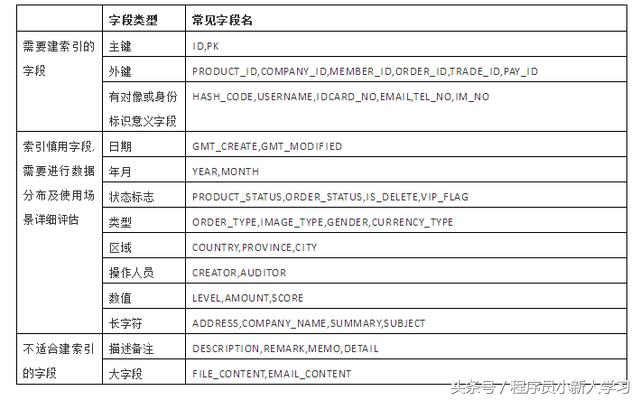# 2、应尽量避免在 where 子句中对字段进行 null 值判断，否则将导致引擎放弃使用索引而进行全表扫描

select id from t where num is null

select id from t where num = 0

1. SELECT ex_question_junior.QUESTION_ID
2. FROM ex_question_junior
3. WHERE IS_USE is NULL

1. SELECT ex_question_junior.QUESTION_ID
2. FROM ex_question_junior
3. WHERE IS_USE =0

# 3、应尽量避免在 where 子句中使用 != 或 <> 操作符，否则将引擎放弃使用索引而进行全表扫描。

1. EXPLAIN
2. SELECT ex_question_junior.QUESTION_ID
3. FROM ex_question_junior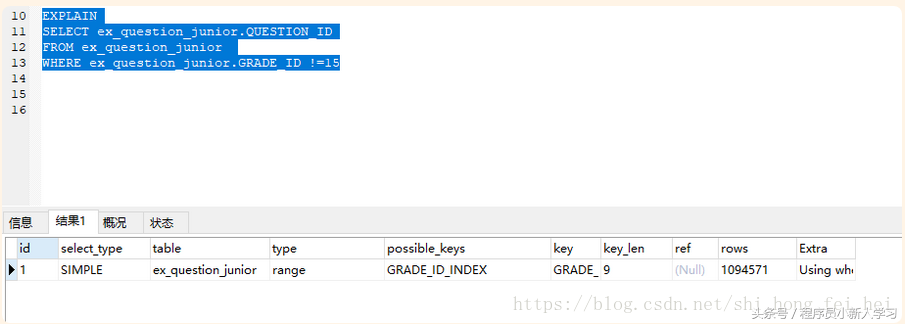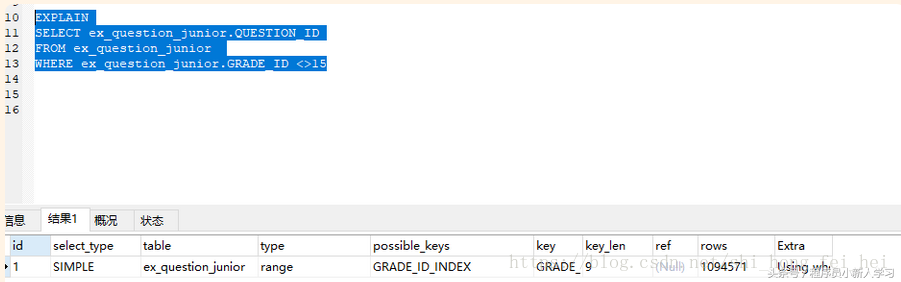# 4.应尽量避免在 where 子句中使用 or 来连接条件，如果一个字段有索引，一个字段没有索引，将导致引擎放弃使用索引而进行全表扫描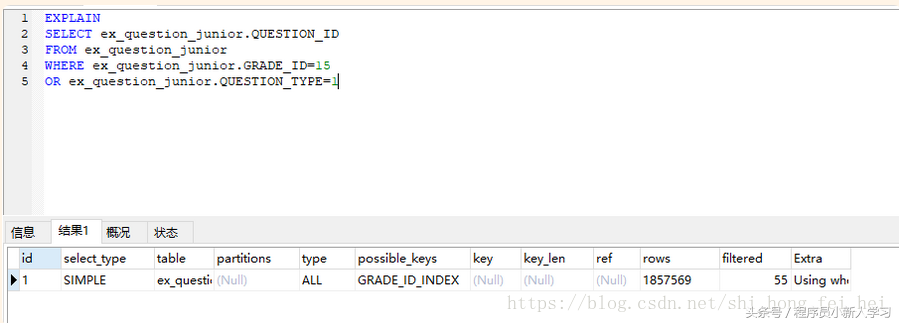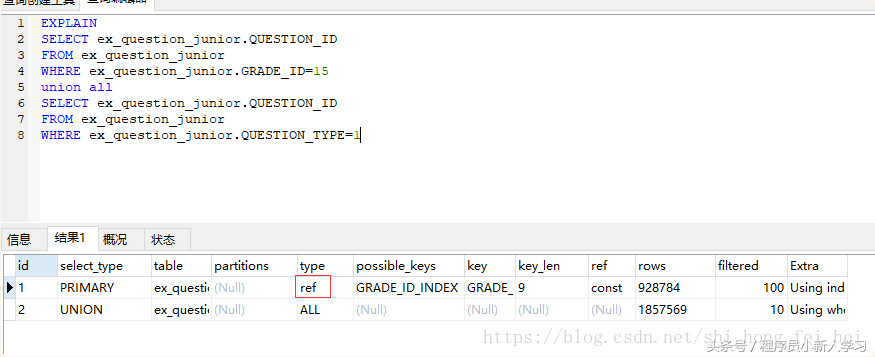# 5.in 和 not in 也要慎用，否则会导致全表扫描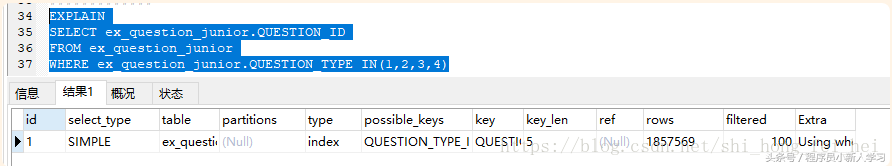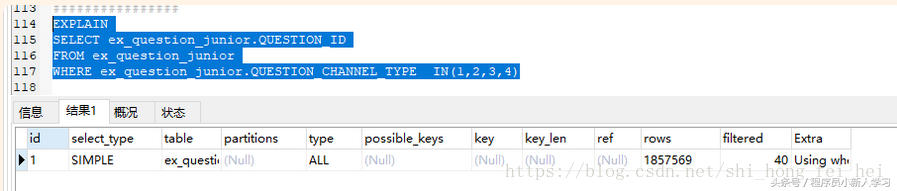1. SELECT ex_question_junior.QUESTION_ID
2. FROM ex_question_junior
3. WHERE ex_question_junior.QUESTION_TYPE IN(1,2,3,4)

1. SELECT ex_question_junior.QUESTION_ID
2. FROM ex_question_junior
3. WHERE ex_question_junior.QUESTION_TYPE between 1 and 4

select * from A

where id in(select id from B)

ex_question_r_knowledge表数据量大，ex_subject_point表数据量小

****************************************************************************

1. SELECT *
2. FROM ex_question_r_knowledge
3. WHERE ex_question_r_knowledge.SUBJECT_POINT_ID IN
4. (
5. SELECT ex_subject_point.SUBJECT_POINT_ID
6. FROM ex_subject_point
7. WHERE ex_subject_point.SUBJECT_ID=7
8. )

1. SELECT *
2. FROM ex_question_r_knowledge
3. WHERE exists
4. (
5. SELECT 1
6. FROM ex_subject_point
7. WHERE ex_subject_point.SUBJECT_ID=7
8. AND ex_subject_point.SUBJECT_POINT_ID = ex_question_r_knowledge.SUBJECT_POINT_ID
9. )

SELECT *

FROM ex_question_r_knowledge

WHERE exists

(

SELECT 1

FROM ex_subject_point

WHERE ex_subject_point.SUBJECT_ID=7

AND ex_subject_point.SUBJECT_POINT_ID = ex_question_r_knowledge.SUBJECT_POINT_ID

)

*************************************************************************

1. SELECT *
2. FROM ex_subject_point
3. WHERE
4. ex_subject_point.SUBJECT_POINT_ID IN( SELECT
5. ex_question_r_knowledge.SUBJECT_POINT_ID FROM
6. ex_question_r_knowledge WHERE

SELECT * FROM ex_subject_point WHERE

ex_subject_point.SUBJECT_POINT_ID IN( SELECT

ex_question_r_knowledge.SUBJECT_POINT_ID FROM

ex_question_r_knowledge WHERE

SELECT *

FROM ex_subject_point

WHERE exists(

SELECT ex_question_r_knowledge.SUBJECT_POINT_ID

FROM ex_question_r_knowledge

AND ex_question_r_knowledge.SUBJECT_POINT_ID= ex_subject_point.SUBJECT_POINT_ID

)

# 6、like模糊全匹配也将导致全表扫描

1. EXPLAIN
2. SELECT *
3. FROM ex_subject_point
4. WHERE ex_subject_point.path like "%/11/%"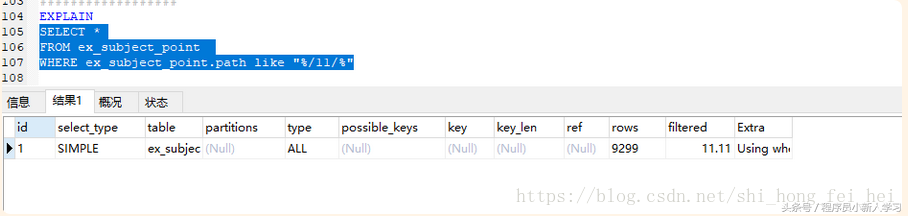like模糊后匹配，不会导致全表扫描

like模糊前匹配，会导致全表扫描

MY SQL的原理就是这样的，LIKE模糊全匹配会导致索引失效，进行全表扫描；LIKE模糊前匹配也会导致索引失效，进行全表扫描；但是LIKE模糊后匹配，索引就会有效果。